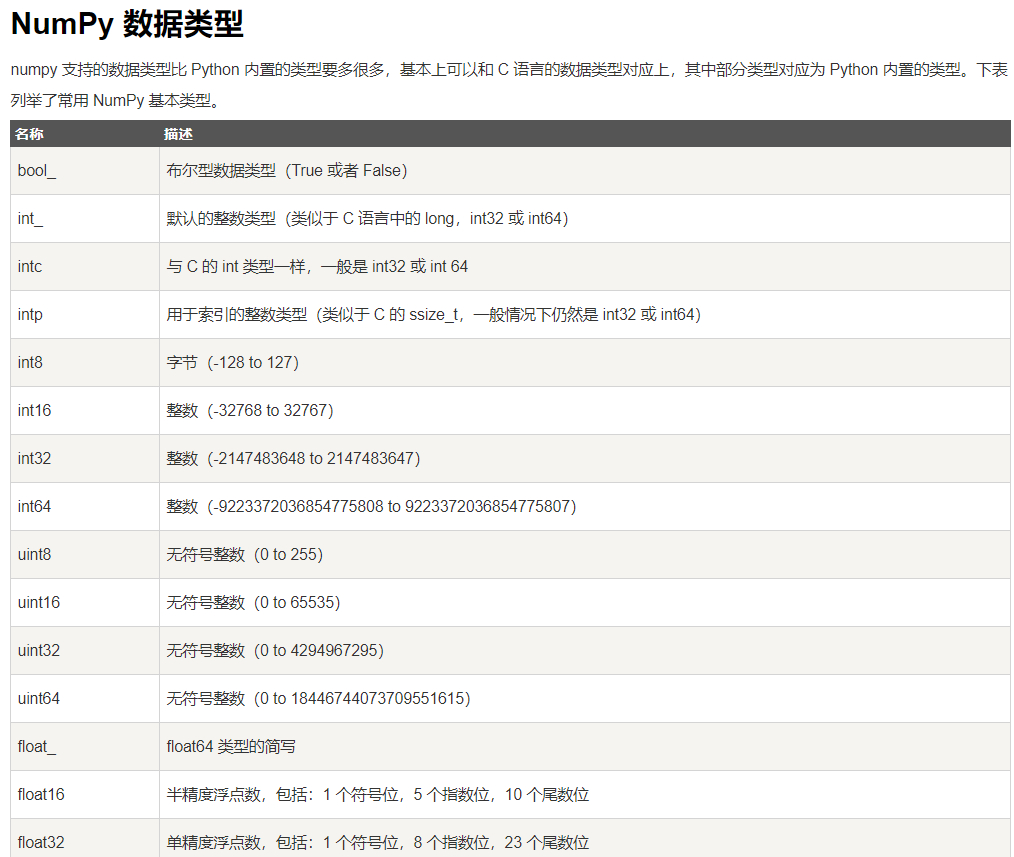Python 的整数与 Numpy 的数据溢出 – Python量化投资

# Python 的整数与 Numpy 的数据溢出• Python 3 中整数的上限是多少？Python 2 呢？
• Numpy 中整数的上限是多少？出现整数溢出该怎么办？

• 一种是短整数，也即常说的整数，用 int 表示，有个内置函数 int()。其大小有限，可通过`sys.maxint()` 查看（取决于平台是 32 位还是 64 位）
• 一种是长整数，即大小无限的整数，用 long 表示，有个内置函数 long()。写法上是在数字后面加大写字母 L 或小写的 l，如 1000L

PEP-237（Unifying Long Integers and Integers）中对这个转变作了说明。它解释这样做的 目的：

Python 在语言运用层屏蔽了很多琐碎的活，比如内存分配，所以，我们在使用字符串、列表或字典等对象时，根本不用操心。整数类型的转变，也是出于这样的便利目的。（坏处是牺牲了一些效率，在此就不谈了）

``````import numpy as np
a = np.arange(2)
type(a)
# 结果：numpy.int32``````

Numpy 支持的数据类型要比 Python 的多，相互间的区分界限很多样：``````import numpy as np
q = 
w = 
# 一个溢出的例子：
a = np.array(q)
b = np.array(w)
print(a*b)  # 产生溢出，结果是个奇怪的数值
# 一个解决的例子：
c = np.array(q, dtype='int64')
d = np.array(w, dtype='int64')
print(c*d) # 没有溢出：``````

• Python 3 极大地简化了整数的表示，效果可表述为：整数就只有一种整数（int），没有其它类型的整数（long、int8、int64 之类的）
• Numpy 中的整数类型对应于 C 语言的数据类型，每种“整数”有自己的区间，要解决数据溢出问题，需要指定更大的数据类型（dtype）https://www.cnblogs.com/pythonista/p/11503117.html

「点点赞赏，手留余香」

还没有人赞赏，快来当第一个赞赏的人吧！
Python
Python
0 条回复 A 作者 M 管理员
所有的伟大，都源于一个勇敢的开始！